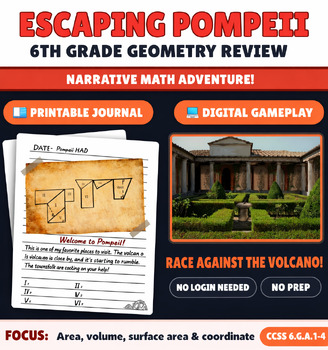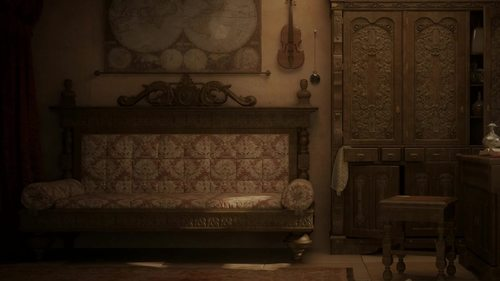# Escaping Pompeii- 6th Grade Geometry Unit ReviewSubject
Resource Type
File Type

Zip

(20 MB)
Product Rating
Standards
• Product Description
• StandardsNEW

You are dropped into Pompeii in 79AD and need to escape before the volcano erupts! This game was created with real Pompeii structures and complements the 6th grade history curriculum. It is created for advanced students meshing problem solving with the geometry common core objectives.

Materials Needed:

• print the journal for recording answers
• laptop or desktop computer

Math Topics Covered:

CCSS.MATH.CONTENT.6.G.A.1

Find the area of right triangles, other triangles, special quadrilaterals, and polygons by composing into rectangles or decomposing into triangles and other shapes; apply these techniques in the context of solving real-world and mathematical problems.

CCSS.MATH.CONTENT.6.G.A.2

Find the volume of a right rectangular prism with fractional edge lengths by packing it with unit cubes of the appropriate unit fraction edge lengths, and show that the volume is the same as would be found by multiplying the edge lengths of the prism. Apply the formulas V = l w h and V = b h to find volumes of right rectangular prisms with fractional edge lengths in the context of solving real-world and mathematical problems.

CCSS.MATH.CONTENT.6.G.A.3

Draw polygons in the coordinate plane given coordinates for the vertices; use coordinates to find the length of a side joining points with the same first coordinate or the same second coordinate. Apply these techniques in the context of solving real-world and mathematical problems.

CCSS.MATH.CONTENT.6.G.A.4

Represent three-dimensional figures using nets made up of rectangles and triangles, and use the nets to find the surface area of these figures. Apply these techniques in the context of solving real-world and mathematical problems.

Other Games in the Series:

This is the second game in the house on the lake series. If you would like to start at the beginning, check out the Escaping Egypt game here. This game has puzzles for 6th grade algebra unit review.

If you would like to try before you buy we have a free version of this game here!

https://www.teacherspayteachers.com/Product/Escaping-Pompeii-Freebie-Area-of-composite-figures-online-math-game-4712810

Feedback:

I always appreciate feedback. Message me if you have any questions and rate my game to let others know how you enjoyed it! Thank you!

Represent three-dimensional figures using nets made up of rectangles and triangles, and use the nets to find the surface area of these figures. Apply these techniques in the context of solving real-world and mathematical problems.
Draw polygons in the coordinate plane given coordinates for the vertices; use coordinates to find the length of a side joining points with the same first coordinate or the same second coordinate. Apply these techniques in the context of solving real-world and mathematical problems.
Find the volume of a right rectangular prism with fractional edge lengths by packing it with unit cubes of the appropriate unit fraction edge lengths, and show that the volume is the same as would be found by multiplying the edge lengths of the prism. Apply the formulas 𝘝 = 𝘭 𝘸 𝘩 and 𝘝 = 𝘣 𝘩 to find volumes of right rectangular prisms with fractional edge lengths in the context of solving real-world and mathematical problems.
Find the area of right triangles, other triangles, special quadrilaterals, and polygons by composing into rectangles or decomposing into triangles and other shapes; apply these techniques in the context of solving real-world and mathematical problems.
Total Pages
N/A
Included
Teaching Duration
90 minutes
Report this Resource to TpT
Reported resources will be reviewed by our team. Report this resource to let us know if this resource violates TpT’s content guidelines.